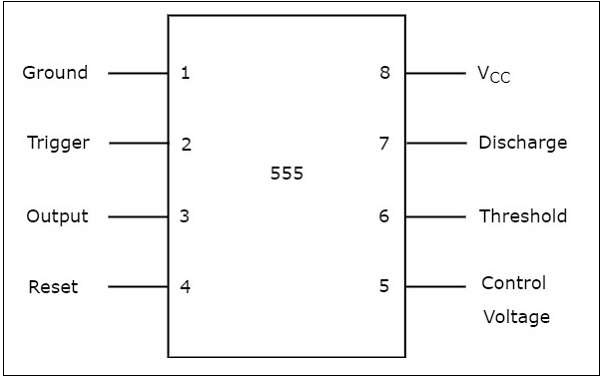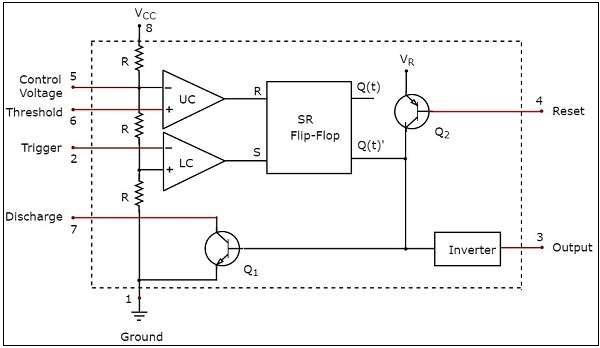# 555 Timer

The 555 Timer IC got its name from the three $5K\Omega$ resistors that are used in its voltage divider network. This IC is useful for generating accurate time delays and oscillations. This chapter explains about 555 Timer in detail.

## Pin Diagram and Functional Diagram

In this section, first let us discuss about the pin diagram of 555 Timer IC and then its functional diagram.

### Pin Diagram

The 555 Timer IC is an 8 pin mini Dual-Inline Package (DIP). The pin diagram of a 555 Timer IC is shown in the following figure −The significance of each pin is self-explanatory from the above diagram. This 555 Timer IC can be operated with a DC supply of +5V to +18V. It is mainly useful for generating non-sinusoidal wave forms like square, ramp, pulse & etc

### Functional Diagram

The pictorial representation showing the internal details of a 555 Timer is known as functional diagram.

The functional diagram of 555 Timer IC is shown in the following figure −Observe that the functional diagram of 555 Timer contains a voltage divider network, two comparators, one SR flip-flop, two transistors and an inverter. This section discusses about the purpose of each block or component in detail −

### Voltage Divider Network

• The voltage divider network consists of a three $5K\Omega$ resistors that are connected in series between the supply voltage $V_{cc}$ and ground.

• This network provides a voltage of $\frac{V_{cc} }{3}$ between a point and ground, if there exists only one $5K\Omega$ resistor. Similarly, it provides a voltage of $\frac{2V_{cc} }{3}$ between a point and ground, if there exists only two $5K\Omega$ resistors.

### Comparator

• The functional diagram of a 555 Timer IC consists of two comparators: an Upper Comparator (UC) and a Lower Comparator (LC).

• Recall that a comparator compares the two inputs that are applied to it and produces an output.

• If the voltage present at the non-inverting terminal of an op-amp is greater than the voltage present at its inverting terminal, then the output of comparator will be $+V_{sat}$. This can be considered as Logic High ('1') in digital representation.

• If the voltage present at the non-inverting terminal of op-amp is less than or equal to the voltage at its inverting terminal, then the output of comparator will be $-V_{sat}$. This can be considered as Logic Low ('0') in digital representation.

### SR Flip-Flop

• Recall that a SR flip-flop operates with either positive clock transitions or negative clock transitions. It has two inputs: S and R, and two outputs: Q(t) and Q(t)’. The outputs, Q(t) & Q(t)’ are complement to each other.

• The following table shows the state table of a SR flip-flop

S R Q(t+1)
0 0 Q(t)
0 1 0
1 0 1
1 1 -
• Here, Q(t) & Q(t+1) are present state & next state respectively. So, SR flip-flop can be used for one of these three functions such as Hold, Reset & Set based on the input conditions, when positive (negative) transition of clock signal is applied.

• The outputs of Lower Comparator (LC) and Upper Comparator (UC) are applied as inputs of SR flip-flop as shown in the functional diagram of 555 Timer IC.

### Transistors and Inverter

• The functional diagram of a 555 Timer IC consists of one npn transistor $Q_{1}$ and one pnp transistor $Q_{2}$. The npn transistor $Q_{1}$ will be turned ON if its base to emitter voltage is positive and greater than cut-in voltage. Otherwise, it will be turned-OFF.

• The pnp transistor $Q_{2}$ is used as buffer in order to isolate the reset input from SR flip-flop and npn transistor $Q_{1}$.

• The inverter used in the functional diagram of a 555 Timer IC not only performs the inverting action but also amplifies the power level.

The 555 Timer IC can be used in mono stable operation in order to produce a pulse at the output. Similarly, it can be used in astable operation in order to produce a square wave at the output.